Imagination is more important than knowledge... Albert Einstein

Guess is more important than calculation --- Knowhowacademy.com

Length - Pythagorean Theorem

For SAT1
Number of Total Problems: 22.
FOR PRINT ::: (Book)

 Problem Num : 1 Type: None Section:Length Theme: Adjustment# : 0 Difficulty: 2Category Pythagorean Theorem
Analysis

 Problem Num : 2 Type: Application Section:Length Theme: Adjustment# : 0 Difficulty: 1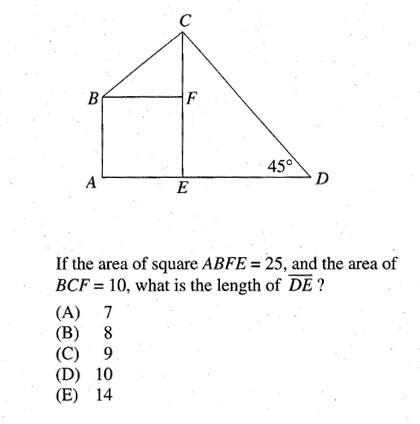Category Pythagorean Theorem
Analysis Length Problem

 Problem Num : 3 Type: None Section:Length Theme: Adjustment# : 1 Difficulty: 1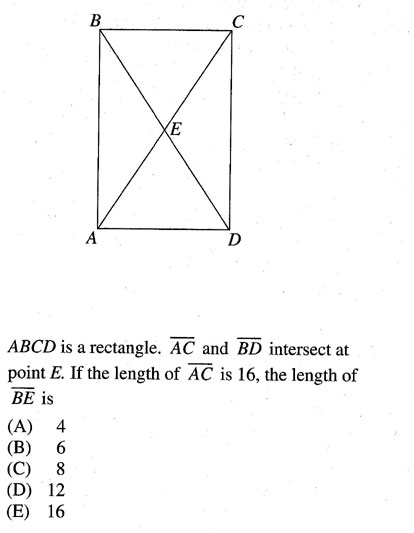Category Pythagorean Theorem
Analysis

 Problem Num : 4 Type: None Section:Length Theme: Adjustment# : 1 Difficulty: 1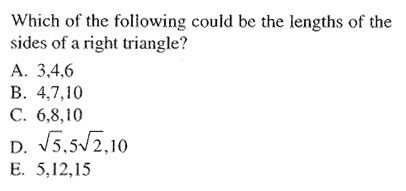Category Pythagorean Theorem
Analysis

 Problem Num : 5 Type: None Section:Length Theme: Adjustment# : 2 Difficulty: 1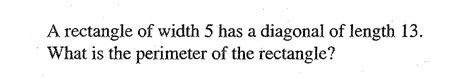Category Pythagorean Theorem
Analysis

 Problem Num : 6 Type: None Section:Length Theme: Adjustment# : 3 Difficulty: 1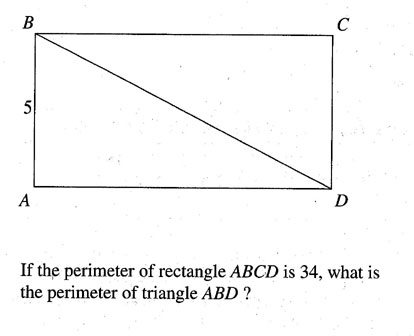Category Pythagorean Theorem
Analysis

 Problem Num : 7 Type: Understanding Section:Length Theme: Adjustment# : 3 Difficulty: 1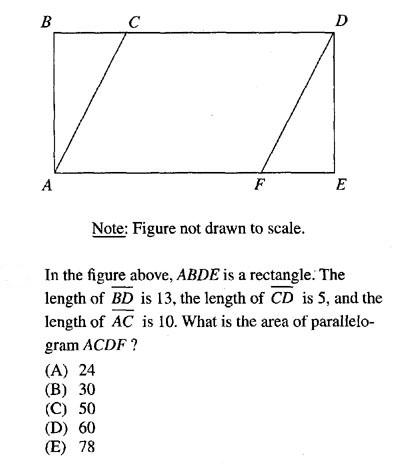Category Pythagorean Theorem
Analysis

 Problem Num : 8 Type: None Section:Length Theme: Adjustment# : 3 Difficulty: 2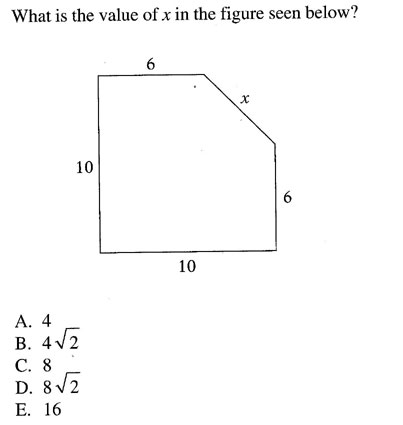Category Pythagorean Theorem
Analysis

 Problem Num : 9 Type: None Section:Length Theme: Adjustment# : 3 Difficulty: 1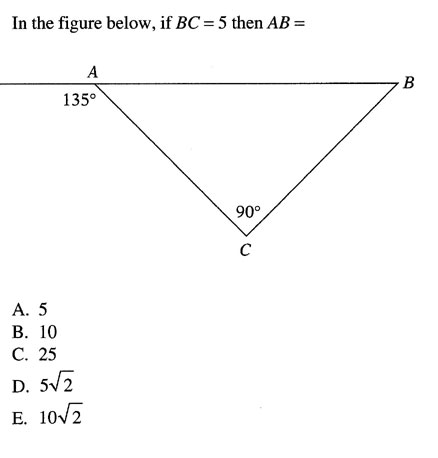Category Pythagorean Theorem
Analysis

 Problem Num : 10 Type: None Section:Length Theme: Adjustment# : 4 Difficulty: 2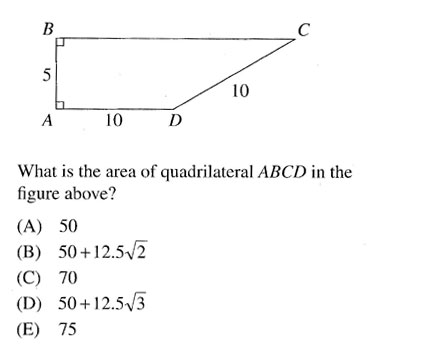Category Pythagorean Theorem
Analysis